# Eighteen Sound

Eighteen Sound is a designer and manufacturer of high quality professional audio loudspeakers, with advanced development and manufacturing technologies, and located in Reggio Emilia, Italy.ound

## no longer sold## Eighteen Sound 1" Driver HD 120

order no. not-available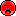- not available

44 mm driver with high quality cone.

• power handling (continuous/programme) = 25 W
• frequency range = 2000-18000 Hz
• resonance frequency fs = 2100 Hz
• impedance R = 8 Ohm
• sound pressure level SPL = 108 dB (2,83V; 1m)
• DC resistance Re = 5,7 Ohm
• voice coil diameter = 44 mm
PDF data sheet## Eighteen Sound 1" Driver/Horn 90 x 60 - XD 120

order no. not-available- not available

44 mm driver with high quality cone.

• power handling (continuous/programme) = 25 W
• frequency range = 2000-18000 Hz
• resonance frequency fs = 2100 Hz
• impedance R = 8 Ohm
• sound pressure level SPL = 108 dB (2,83V; 1m)
• DC resistance Re = 5,7 Ohm
• voice coil diameter = 44 mm
• mounting diameter d = 150x200 mm
• mounting depth (not countersunk) t = 150 mm
PDF data sheet## Eighteen Sound Neodym Driver ND 1060

order no. not-available- not available

44 mm driver with high quality cone. The magnet system is based on Neodymium technology.

• power handling (continuous/programme) = 40 W
• frequency range = 1600-20000 Hz
• resonance frequency fs = 780 Hz
• impedance R = 8 Ohm
• sound pressure level SPL = 108 dB (2,83V; 1m)
• DC resistance Re = 6,2 Ohm
• voice coil diameter = 44 mm## Eighteen Sound 1" Neodym Driver ND 1080

order no. not-available- not available

44 mm driver with high quality cone. The magnet system is based on Neodymium technology.

• power handling (continuous/programme) = 50 W
• frequency range = 1600-20000 Hz
• resonance frequency fs = 980 Hz
• impedance R = 8 Ohm
• sound pressure level SPL = 108 dB (2,83V; 1m)
• DC resistance Re = 6,2 Ohm
• voice coil diameter = 44 mm
PDF data sheet

## Eighteen Sound Titanium Driver ND 4015 T

order no. not-available- not available

1.5 inch high frequency compression driver with titanium cone. The magnet system is based on Neodymium technology.

• power handling (continuous/programme) = 160/320 W
• frequency range = 800-20000 Hz
• resonance frequency fs = 400 Hz
• impedance R = 8 Ohm
• sound pressure level SPL = 111 dB (2,83V; 1m)
• DC resistance Re = 6 Ohm
• force factor BL = 14,1 N/A
• voice coil diameter = 100 mm
• mass m = 3,2 kg
PDF data sheet

## Eighteen Sound Neodym Driver NSD 4015 N

order no. not-available- not available

1.5 inch high frequency compression driver with titanium cone. The magnet system is based on Neodymium technology.

• power handling (continuous/programme) = 160/320 W
• frequency range = 800-20000 Hz
• resonance frequency fs = 390 Hz
• impedance R = 8 Ohm
• sound pressure level SPL = 111 dB (2,83V; 1m)
• DC resistance Re = 6 Ohm
• force factor BL = 17 N/A
• voice coil diameter = 100 mm
• mass m = 3,2 kg
PDF data sheet

## Eighteen Sound XR 1564

order no. not-available- not available

Horn for a compression driver with 1,5 inch throat.

• Throat diameter = 1,5 inch
• nominal angle (HxV) = 60x40 Deg
• Cut off frequency : 800 Hz
• over all dimensions (HxWxD) = 270 x 270 x 180 mm
• baffle cutout dimensions (HxW) = 250 x 250 mm
• net weight = 1,9 kg
PDF data sheet

## Eighteen Sound XR 1464

order no. not-available- not available

Horn for a compression driver with 1,4 inch throat.

• Throat diameter = 1,4 inch
• nominal angle (HxV) = 60x40 Deg
• Cut off frequency : 800 Hz
• over all dimensions (HxWxD) = 270 x 270 x 180 mm
• baffle cutout dimensions (HxW) = 250 x 250 mm
• net weight = 1,9 kg
PDF data sheet

## Eighteen Sound XR 1496

order no. not-available- not available

Horn for a compression driver with 1,4 inch throat.

• Throat diameter = 1,4 inch
• nominal angle (HxV) = 90x60 Deg
• Cut off frequency : 800 Hz
• over all dimensions (HxWxD) = 270 x 270 x 180 mm
• baffle cutout dimensions (HxW) = 250 x 250 mm
• net weight = 1,9 kg
PDF data sheet

## Eighteen Sound XR 2064

order no. not-available- not available

Horn for a compression driver with 2 inch throat.

• Throat diameter = 2 inch
• nominal angle (HxV) = 60x40 Deg
• Cut off frequency : 800 Hz
• over all dimensions (HxWxD) = 270 x 270 x 200 mm
• baffle cutout dimensions (HxW) = 250 x 250 mm
• net weight = 2 kg
PDF data sheetopen a bigger photo

recommended cabinet 1:
closed cabinet with 0,53 L volume
from 396/250 Hz (-3dB/-8dB)

recommended cabinet 2:
1,2 L volume bass reflex cabinet
with HP50 reflex tube, 8 cm long.
from 240/180 Hz (-3dB/-8dB).

## Eighteen Sound 6 ND 410

order no. not-available- not available

15 cm midrange driver with paper cone. The basket is made of die-cast. The magnet system is based on Neodymium technology.

• power handling (continuous/programme) = 240/480 W
• frequency range = 200-8000 Hz
• resonance frequency fs = 120 Hz
• impedance R = 8 Ohm
• sound pressure level SPL = 102 dB (2,83V; 1m)
• DC resistance Re = 5,9 Ohm
• force factor BL = 11,6 N/A
• voice coil inductance L = 0,67 mH
• effective piston radiating area Sd = 143 cm2
• effective mechanical mass incl. air load mms = 8,2 g
• equivalent volume of compliance Vas = 6,2 l
• total Q factor Qts = 0,24 (Qms=2,2, Qes=0,27)
• voice coil diameter = 44 mm
• maximum peak linear excursion vibration xlin = +/- 2 mm
• mounting diameter d = 148 mm
• overall diameter d = 162 mm
• mounting depth (not countersunk) t = 60 mm
• mass m = 1,25 kg
PDF data sheet

## Eighteen Sound 6 ND 410, 16 Ohm

order no. not-available- not available

15 cm midrange driver with paper cone. The basket is made of die-cast. The magnet system is based on Neodymium technology.

• power handling (continuous/programme) = 240/480 W
• impedance R = 16 Ohm
• effective piston radiating area Sd = 143 cm2
• voice coil diameter = 44 mm
• mounting diameter d = 148 mm
• overall diameter d = 162 mm
• mounting depth (not countersunk) t = 60 mm
• mass m = 1,25 kg
PDF data sheet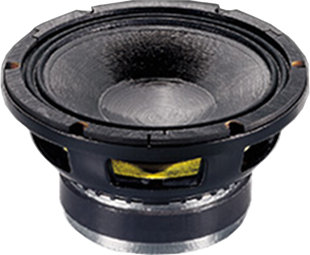## Eighteen Sound 8 MB 400, 4 Ohm

order no. not-available- not available

20 cm bass-midrange driver with paper cone. The basket is made of die-cast.

• power handling (continuous/programme) = 280/400 W
• frequency range = 55-5200 Hz
• impedance R = 4 Ohm
• effective piston radiating area Sd = 227 cm2
• voice coil diameter = 51 mm
• mounting diameter d = 185 mm
• overall diameter d = 210 mm
• mounting depth (not countersunk) t = 101 mm
• mass m = 3,6 kg
PDF data sheetopen a bigger photo

recommended cabinet 1:
closed cabinet with 10,1 L volume
from 137/86 Hz (-3dB/-8dB)

recommended cabinet 2:
33 L volume bass reflex cabinet
with 2x HP100 reflex tube, 17 cm long.
from 72/56 Hz (-3dB/-8dB).

## Eighteen Sound 12 W 700

order no. not-available- not available

30 cm bass driver with paper cone. The basket is made of die-cast.

• power handling (continuous/programme) = 450/700 W
• frequency range = 55-4200 Hz
• resonance frequency fs = 58 Hz
• impedance R = 8 Ohm
• sound pressure level SPL = 98 dB (2,83V; 1m)
• DC resistance Re = 5,7 Ohm
• force factor BL = 17,7 N/A
• voice coil inductance L = 1,48 mH
• effective piston radiating area Sd = 531 cm2
• effective mechanical mass incl. air load mms = 51 g
• equivalent volume of compliance Vas = 55 l
• total Q factor Qts = 0,34 (Qms=3,93, Qes=0,37)
• voice coil diameter = 75 mm
• maximum peak linear excursion vibration xlin = +/- 6,5 mm
• mounting diameter d = 282 mm
• overall diameter d = 315 mm
• mounting depth (not countersunk) t = 148 mm
• mass m = 8,2 kg
PDF data sheetopen a bigger photo

recommended cabinet 1:
closed cabinet with 12,4 L volume
from 151/95 Hz (-3dB/-8dB)

recommended cabinet 2:
34 L volume bass reflex cabinet
with 2x HP100 reflex tube, 12 cm long.
from 86/65 Hz (-3dB/-8dB).

## Eighteen Sound 12 NMB 420

order no. not-available- not available

30 cm bass driver with paper cone. The basket is made of die-cast. The magnet system is based on Neodymium technology.

• power handling (continuous/programme) = 450/900 W
• frequency range = 55-6000 Hz
• resonance frequency fs = 53 Hz
• impedance R = 8 Ohm
• sound pressure level SPL = 100,5 dB (2,83V; 1m)
• DC resistance Re = 5,2 Ohm
• force factor BL = 13,9 N/A
• voice coil inductance L = 0,2 mH
• effective piston radiating area Sd = 530 cm2
• effective mechanical mass incl. air load mms = 33,5 g
• equivalent volume of compliance Vas = 105 l
• total Q factor Qts = 0,28 (Qms=3,6, Qes=0,3)
• voice coil diameter = 65 mm
• maximum peak linear excursion vibration xlin = +/- 4 mm
• mounting diameter d = 282 mm
• overall diameter d = 315 mm
• mounting depth (not countersunk) t = 127 mm
• mass m = 2,8 kg
PDF data sheet

## Eighteen Sound 12 LW 801, 4 Ohm

order no. not-available- not available

30 cm bass driver with paper cone. The basket is made of die-cast.

• power handling (continuous/programme) = 500/800 W
• frequency range = 40-4000 Hz
• impedance R = 4 Ohm
• effective piston radiating area Sd = 531 cm2
• voice coil diameter = 75 mm
• mounting diameter d = 282 mm
• overall diameter d = 315 mm
• mounting depth (not countersunk) t = 149 mm
• mass m = 8,1 kg
PDF data sheet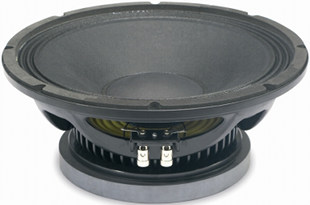open a bigger photo

recommended cabinet 1:
closed cabinet with 6,4 L volume
from 172/109 Hz (-3dB/-8dB)

recommended cabinet 2:
13,5 L volume bass reflex cabinet
with HP100 reflex tube, 16 cm long.
from 108/81 Hz (-3dB/-8dB).

## Eighteen Sound 12 MB 650

order no. not-available- not available

30 cm bass driver with paper cone. The basket is made of die-cast.

• power handling (continuous/programme) = 800/800 W
• frequency range = 45-5000 Hz
• resonance frequency fs = 48 Hz
• impedance R = 8 Ohm
• sound pressure level SPL = 98 dB (2,83V; 1m)
• DC resistance Re = 6 Ohm
• force factor BL = 19 N/A
• voice coil inductance L = 0,83 mH
• effective piston radiating area Sd = 530 cm2
• effective mechanical mass incl. air load mms = 48 g
• equivalent volume of compliance Vas = 90 l
• total Q factor Qts = 0,22 (Qms=3,2, Qes=0,24)
• voice coil diameter = 65 mm
• maximum peak linear excursion vibration xlin = +/- 5,5 mm
• mounting diameter d = 280 mm
• overall diameter d = 310 mm
• mounting depth (not countersunk) t = 143 mm
• mass m = 6,8 kg
PDF data sheetopen a bigger photo

recommended cabinet 1:
closed cabinet with 20 L volume
from 121/76 Hz (-3dB/-8dB)

recommended cabinet 2:
57 L volume bass reflex cabinet
with 2x HP100 reflex tube, 11 cm long.
from 68/52 Hz (-3dB/-8dB).

## Eighteen Sound 15 ND 730

order no. not-available- not available

37 cm bass driver with paper cone. The basket is made of die-cast. The magnet system is based on Neodymium technology.

• power handling (continuous/programme) = 400/600 W
• frequency range = 44-4000 Hz
• resonance frequency fs = 44 Hz
• impedance R = 8 Ohm
• sound pressure level SPL = 99 dB (2,83V; 1m)
• DC resistance Re = 5,5 Ohm
• force factor BL = 21 N/A
• voice coil inductance L = 1,35 mH
• effective piston radiating area Sd = 850 cm2
• effective mechanical mass incl. air load mms = 86 g
• equivalent volume of compliance Vas = 156 l
• total Q factor Qts = 0,29 (Qms=8,1, Qes=0,3)
• voice coil diameter = 75 mm
• maximum peak linear excursion vibration xlin = +/- 6,5 mm
• mounting diameter d = 353 mm
• overall diameter d = 387 mm
• mounting depth (not countersunk) t = 169,5 mm
• mass m = 4,8 kg
PDF data sheetopen a bigger photo

recommended cabinet 1:
closed cabinet with 13,9 L volume
from 119/75 Hz (-3dB/-8dB)

recommended cabinet 2:
38 L volume bass reflex cabinet
with 2x HP100 reflex tube, 22 cm long.
from 68/52 Hz (-3dB/-8dB).

## Eighteen Sound 15 NLW 9400

order no. not-available- not available

37 cm bass driver with paper cone. The basket is made of die-cast. The magnet system is based on Neodymium technology.

• power handling (continuous/programme) = 1000/1400 W
• frequency range = 40-4000 Hz
• resonance frequency fs = 42 Hz
• impedance R = 8 Ohm
• sound pressure level SPL = 97 dB (2,83V; 1m)
• DC resistance Re = 5,2 Ohm
• force factor BL = 25 N/A
• voice coil inductance L = 1,1 mH
• effective piston radiating area Sd = 900 cm2
• effective mechanical mass incl. air load mms = 131 g
• equivalent volume of compliance Vas = 118 l
• total Q factor Qts = 0,28 (Qms=10,4, Qes=0,29)
• voice coil diameter = 100 mm
• maximum peak linear excursion vibration xlin = +/- 9,5 mm
• mounting diameter d = 353 mm
• overall diameter d = 387 mm
• mounting depth (not countersunk) t = 174 mm
• mass m = 6,8 kg
PDF data sheetopen a bigger photo

recommended cabinet 1:
closed cabinet with 49 L volume
from 85/54 Hz (-3dB/-8dB)

recommended cabinet 2:
129 L volume bass reflex cabinet
with 4x HP100 reflex tube, 28 cm long.
from 49/37 Hz (-3dB/-8dB).

## Eighteen Sound 18 LW 800

order no. not-available- not available

44 cm bass driver with paper cone. The basket is made of die-cast.

• power handling (continuous/programme) = 500/600 W
• frequency range = 35-3300 Hz
• resonance frequency fs = 29 Hz
• impedance R = 8 Ohm
• sound pressure level SPL = 99,5 dB (2,83V; 1m)
• DC resistance Re = 5 Ohm
• force factor BL = 20 N/A
• voice coil inductance L = 1,94 mH
• effective piston radiating area Sd = 1133 cm2
• effective mechanical mass incl. air load mms = 123 g
• equivalent volume of compliance Vas = 448 l
• total Q factor Qts = 0,27 (Qms=4,5, Qes=0,29)
• voice coil diameter = 75 mm
• maximum peak linear excursion vibration xlin = +/- 8 mm
• mounting diameter d = 416 mm
• overall diameter d = 462 mm
• mounting depth (not countersunk) t = 200 mm
• mass m = 9,1 kg
PDF data sheetopen a bigger photo

recommended cabinet 1:
closed cabinet with 21 L volume
from 120/76 Hz (-3dB/-8dB)

recommended cabinet 2:
46 L volume bass reflex cabinet
with 2x HP100 reflex tube, 16 cm long.
from 74/55 Hz (-3dB/-8dB).

## Eighteen Sound 18 W 1000

order no. not-available- not available

44 cm bass driver with paper cone. The basket is made of die-cast.

• power handling (continuous/programme) = 1000/1400 W
• frequency range = 37-4700 Hz
• resonance frequency fs = 35 Hz
• impedance R = 8 Ohm
• sound pressure level SPL = 99 dB (2,83V; 1m)
• DC resistance Re = 5,8 Ohm
• force factor BL = 27,6 N/A
• voice coil inductance L = 2,45 mH
• effective piston radiating area Sd = 1134 cm2
• effective mechanical mass incl. air load mms = 139 g
• equivalent volume of compliance Vas = 268 l
• total Q factor Qts = 0,23 (Qms=7,1, Qes=0,24)
• voice coil diameter = 100 mm
• maximum peak linear excursion vibration xlin = +/- 7 mm
• mounting diameter d = 416 mm
• overall diameter d = 462 mm
• mounting depth (not countersunk) t = 205,9 mm
• mass m = 12,9 kg
PDF data sheet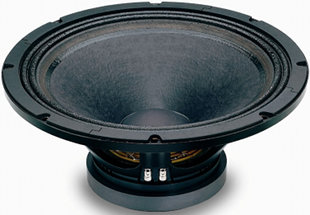open a bigger photo

recommended cabinet 1:
closed cabinet with 29 L volume
from 103/65 Hz (-3dB/-8dB)

recommended cabinet 2:
77 L volume bass reflex cabinet
with 4x HP100 reflex tube, 34 cm long.
from 59/45 Hz (-3dB/-8dB).

## Eighteen Sound 18 LW 1250

order no. not-available- not available

44 cm bass driver with paper cone. The basket is made of die-cast.

• power handling (continuous/programme) = 1000/1400 W
• frequency range = 35-3500 Hz
• resonance frequency fs = 35 Hz
• impedance R = 8 Ohm
• sound pressure level SPL = 98 dB (2,83V; 1m)
• DC resistance Re = 5 Ohm
• force factor BL = 23,6 N/A
• voice coil inductance L = 2,73 mH
• effective piston radiating area Sd = 1134 cm2
• effective mechanical mass incl. air load mms = 142 g
• equivalent volume of compliance Vas = 268 l
• total Q factor Qts = 0,27 (Qms=8, Qes=0,28)
• voice coil diameter = 100 mm
• maximum peak linear excursion vibration xlin = +/- 9 mm
• mounting diameter d = 416 mm
• overall diameter d = 462 mm
• mounting depth (not countersunk) t = 207,9 mm
• mass m = 13 kg
PDF data sheetopen a bigger photo

recommended cabinet 1:
closed cabinet with 60 L volume
from 71/45 Hz (-3dB/-8dB)

recommended cabinet 2:
202 L volume bass reflex cabinet
with 4x HP100 reflex tube, 24 cm long.
from 37/29 Hz (-3dB/-8dB).

## Eighteen Sound 18 ND 9310 B

order no. not-available- not available

44 cm bass driver with paper cone. The basket is made of die-cast. The magnet system is based on Neodymium technology.

• power handling (continuous/programme) = 1000/1400 W
• frequency range = 28-2500 Hz
• resonance frequency fs = 31 Hz
• impedance R = 8 Ohm
• sound pressure level SPL = 98 dB (2,83V; 1m)
• DC resistance Re = 5 Ohm
• force factor BL = 21,8 N/A
• voice coil inductance L = 1,2 mH
• effective piston radiating area Sd = 1220 cm2
• effective mechanical mass incl. air load mms = 182 g
• equivalent volume of compliance Vas = 303 l
• total Q factor Qts = 0,35 (Qms=7, Qes=0,37)
• voice coil diameter = 100 mm
• maximum peak linear excursion vibration xlin = +/- 9,5 mm
• mounting diameter d = 416 mm
• overall diameter d = 462 mm
• mounting depth (not countersunk) t = 223,5 mm
• mass m = 7,6 kg
PDF data sheet

## Eighteen Sound 18 ND 9310 B, 4 Ohm

order no. not-available- not available

44 cm bass driver with paper cone. The basket is made of die-cast. The magnet system is based on Neodymium technology.

• power handling (continuous/programme) = 1000/1400 W
• frequency range = 28-2500 Hz
• impedance R = 4 Ohm
• effective piston radiating area Sd = 1220 cm2
• voice coil diameter = 100 mm
• mounting diameter d = 416 mm
• overall diameter d = 462 mm
• mounting depth (not countersunk) t = 223,5 mm
• mass m = 7,6 kg
PDF data sheet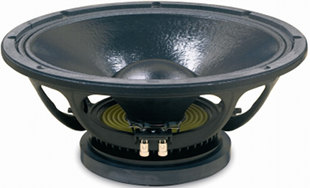open a bigger photo

recommended cabinet 1:
closed cabinet with 17 L volume
from 118/75 Hz (-3dB/-8dB)

recommended cabinet 2:
36 L volume bass reflex cabinet
with 2x HP100 reflex tube, 25 cm long.
from 74/55 Hz (-3dB/-8dB).

## Eighteen Sound 15 W 930

order no. not-available- not available

38 cm bass driver with paper cone. The basket is made of die-cast.

• power handling (continuous/programme) = 500/800 W
• frequency range = 50-3600 Hz
• resonance frequency fs = 33 Hz
• impedance R = 8 Ohm
• sound pressure level SPL = 98 dB (2,83V; 1m)
• DC resistance Re = 5,5 Ohm
• force factor BL = 22,1 N/A
• voice coil inductance L = 1,47 mH
• effective piston radiating area Sd = 860 cm2
• effective mechanical mass incl. air load mms = 97 g
• equivalent volume of compliance Vas = 240 l
• total Q factor Qts = 0,22 (Qms=8,78, Qes=0,23)
• voice coil diameter = 75 mm
• maximum peak linear excursion vibration xlin = +/- 7,5 mm
• mounting diameter d = 360 mm
• overall diameter d = 393 mm
• mounting depth (not countersunk) t = 185 mm
• mass m = 7,6 kg
PDF data sheet

## Eighteen Sound 15 W 930, 4 Ohm

order no. not-available- not available

38 cm bass driver with paper cone. The basket is made of die-cast.

• power handling (continuous/programme) = 500/800 W
• frequency range = 50-3600 Hz
• impedance R = 4 Ohm
• effective piston radiating area Sd = 860 cm2
• voice coil diameter = 75 mm
• mounting diameter d = 360 mm
• overall diameter d = 393 mm
• mounting depth (not countersunk) t = 185 mm
• mass m = 7,6 kg
PDF data sheet

## Eighteen Sound 15 W 930, 16 Ohm

order no. not-available- not available

38 cm bass driver with paper cone. The basket is made of die-cast.

• power handling (continuous/programme) = 500/800 W
• frequency range = 50-3600 Hz
• impedance R = 16 Ohm
• effective piston radiating area Sd = 860 cm2
• voice coil diameter = 75 mm
• mounting diameter d = 360 mm
• overall diameter d = 393 mm
• mounting depth (not countersunk) t = 185 mm
• mass m = 7,6 kg
PDF data sheet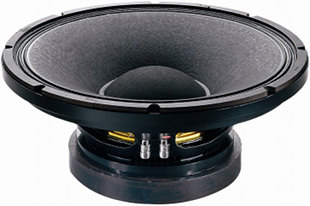open a bigger photo

recommended cabinet 1:
closed cabinet with 12,4 L volume
from 143/90 Hz (-3dB/-8dB)

recommended cabinet 2:
30 L volume bass reflex cabinet
with 2x HP100 reflex tube, 18 cm long.
from 85/64 Hz (-3dB/-8dB).

## Eighteen Sound 15 W 1200

order no. not-available- not available

37 cm bass driver with paper cone. The basket is made of die-cast.

• power handling (continuous/programme) = 850/1200 W
• frequency range = 43-4100 Hz
• resonance frequency fs = 45 Hz
• impedance R = 8 Ohm
• sound pressure level SPL = 99,5 dB (2,83V; 1m)
• DC resistance Re = 5,5 Ohm
• force factor BL = 25 N/A
• voice coil inductance L = 1,7 mH
• effective piston radiating area Sd = 900 cm2
• effective mechanical mass incl. air load mms = 108 g
• equivalent volume of compliance Vas = 134 l
• total Q factor Qts = 0,25 (Qms=4,1, Qes=0,27)
• voice coil diameter = 100 mm
• maximum peak linear excursion vibration xlin = +/- 6 mm
• mounting diameter d = 353 mm
• overall diameter d = 387 mm
• mounting depth (not countersunk) t = 162 mm
• mass m = 12,1 kg
PDF data sheetopen a bigger photo

recommended cabinet 1:
closed cabinet with 119 L volume
from 76/48 Hz (-3dB/-8dB)

recommended cabinet 2:
486 L volume bass reflex cabinet
with 4x HP100 reflex tube, -2 cm long.
from 35/30 Hz (-3dB/-8dB).

## Eighteen Sound 15 W 500

order no. not-available- not available

37 cm bass driver with paper cone. The basket is made of die-cast.

• power handling (continuous/programme) = 350/500 W
• frequency range = 50-4500 Hz
• resonance frequency fs = 50 Hz
• impedance R = 8 Ohm
• sound pressure level SPL = 100,5 dB (2,83V; 1m)
• DC resistance Re = 5,2 Ohm
• force factor BL = 12,6 N/A
• voice coil inductance L = 1,04 mH
• effective piston radiating area Sd = 850 cm2
• effective mechanical mass incl. air load mms = 55 g
• equivalent volume of compliance Vas = 189 l
• total Q factor Qts = 0,52 (Qms=9,64, Qes=0,55)
• voice coil diameter = 64 mm
• maximum peak linear excursion vibration xlin = +/- 4 mm
• mounting diameter d = 353 mm
• overall diameter d = 387 mm
• mounting depth (not countersunk) t = 161 mm
• mass m = 4,3 kg
PDF data sheetopen a bigger photo

recommended cabinet 1:
closed cabinet with 37 L volume
from 94/59 Hz (-3dB/-8dB)

recommended cabinet 2:
102 L volume bass reflex cabinet
with 4x HP100 reflex tube, 30 cm long.
from 53/41 Hz (-3dB/-8dB).

## Eighteen Sound 18 ND 930

order no. not-available- not available

44 cm bass driver with paper cone. The basket is made of die-cast. The magnet system is based on Neodymium technology.

• power handling (continuous/programme) = 500/800 W
• frequency range = 35-3800 Hz
• resonance frequency fs = 33 Hz
• impedance R = 8 Ohm
• sound pressure level SPL = 99 dB (2,83V; 1m)
• DC resistance Re = 5,5 Ohm
• force factor BL = 22 N/A
• voice coil inductance L = 1,7 mH
• effective piston radiating area Sd = 1133 cm2
• effective mechanical mass incl. air load mms = 133 g
• equivalent volume of compliance Vas = 316 l
• total Q factor Qts = 0,28 (Qms=7,65, Qes=0,29)
• voice coil diameter = 75 mm
• maximum peak linear excursion vibration xlin = +/- 7,5 mm
• mounting diameter d = 416 mm
• overall diameter d = 462 mm
• mounting depth (not countersunk) t = 201,5 mm
• mass m = 5,9 kg
PDF data sheetopen a bigger photo

recommended cabinet 1:
closed cabinet with 18 L volume
from 97/61 Hz (-3dB/-8dB)

recommended cabinet 2:
48 L volume bass reflex cabinet
with 2x HP100 reflex tube, 28 cm long.
from 55/42 Hz (-3dB/-8dB).

## Eighteen Sound 18 NLW 9600

order no. not-available- not available

44 cm bass driver with paper cone. The basket is made of die-cast. The magnet system is based on Neodymium technology.

• power handling (continuous/programme) = 1800/3600 W
• frequency range = 32-2500 Hz
• resonance frequency fs = 34 Hz
• impedance R = 8 Ohm
• sound pressure level SPL = 97 dB (2,83V; 1m)
• DC resistance Re = 4,7 Ohm
• force factor BL = 30 N/A
• voice coil inductance L = 2,11 mH
• effective piston radiating area Sd = 1134 cm2
• effective mechanical mass incl. air load mms = 261 g
• equivalent volume of compliance Vas = 149 l
• total Q factor Qts = 0,28 (Qms=8,7, Qes=0,29)
• voice coil diameter = 135 mm
• maximum peak linear excursion vibration xlin = +/- 14 mm
• mounting diameter d = 416 mm
• overall diameter d = 462 mm
• mounting depth (not countersunk) t = 236 mm
• mass m = 12,5 kg
PDF data sheetopen a bigger photo

recommended cabinet 1:
closed cabinet with 67 L volume
from 76/48 Hz (-3dB/-8dB)

recommended cabinet 2:
226 L volume bass reflex cabinet
with 4x HP100 reflex tube, 16 cm long.
from 39/31 Hz (-3dB/-8dB).

## Eighteen Sound 21 NLW 9400

order no. not-available- not available

52 cm bass driver with paper cone. The basket is made of die-cast. The magnet system is based on Neodymium technology.

• power handling (continuous/programme) = 1200/2400 W
• frequency range = 30-1500 Hz
• resonance frequency fs = 33 Hz
• impedance R = 8 Ohm
• sound pressure level SPL = 97,5 dB (2,83V; 1m)
• DC resistance Re = 3,5 Ohm
• force factor BL = 23 N/A
• voice coil inductance L = 1,51 mH
• effective piston radiating area Sd = 1662 cm2
• effective mechanical mass incl. air load mms = 248 g
• equivalent volume of compliance Vas = 340 l
• total Q factor Qts = 0,35 (Qms=8,85, Qes=0,36)
• voice coil diameter = 100 mm
• maximum peak linear excursion vibration xlin = +/- 9,5 mm
• mounting diameter d = 492 mm
• overall diameter d = 545 mm
• mounting depth (not countersunk) t = 240 mm
• mass m = 9,45 kg
PDF data sheet

## Eighteen Sound 21 NLW 9400, 4 Ohm

order no. not-available- not available

52 cm bass driver with paper cone. The basket is made of die-cast. The magnet system is based on Neodymium technology.

• power handling (continuous/programme) = 1200/2400 W
• frequency range = 30-1500 Hz
• impedance R = 4 Ohm
• effective piston radiating area Sd = 1662 cm2
• voice coil diameter = 100 mm
• mounting diameter d = 492 mm
• overall diameter d = 545 mm
• mounting depth (not countersunk) t = 240 mm
• mass m = 9,45 kg
PDF data sheetopen a bigger photo

recommended cabinet 1:
closed cabinet with 5,8 L volume
from 135/85 Hz (-3dB/-8dB)

recommended cabinet 2:
21 L volume bass reflex cabinet
with HP100 reflex tube, 16 cm long.
from 68/54 Hz (-3dB/-8dB).

## Eighteen Sound 8 CX 400 F

order no. not-available- not available

20 cm coax driver with paper cone. The basket is made of die-cast.

• power handling (continuous/programme) = 280/400 W
• frequency range = 65-20000 Hz
• resonance frequency fs = 64 Hz
• impedance R = 8 Ohm
• sound pressure level SPL = 95 dB (2,83V; 1m)
• DC resistance Re = 5 Ohm
• force factor BL = 9,3 N/A
• voice coil inductance L = 0,96 mH
• effective piston radiating area Sd = 227 cm2
• effective mechanical mass incl. air load mms = 18 g
• equivalent volume of compliance Vas = 23,9 l
• total Q factor Qts = 0,38 (Qms=3,23, Qes=0,43)
• voice coil diameter = 51 mm
• maximum peak linear excursion vibration xlin = +/- 5,8 mm
• mounting diameter d = 186 mm
• overall diameter d = 210 mm
• mounting depth (not countersunk) t = 150,5 mm
• mass m = 4,4 kg
PDF data sheetopen a bigger photo

recommended cabinet 1:
closed cabinet with 6,7 L volume
from 168/106 Hz (-3dB/-8dB)

recommended cabinet 2:
16 L volume bass reflex cabinet
with HP100 reflex tube, 13 cm long.
from 100/75 Hz (-3dB/-8dB).

## Eighteen Sound 12 CX 800

order no. not-available- not available

30 cm coax driver with paper cone. The basket is made of die-cast.

• power handling (continuous/programme) = 400/600 W
• frequency range = 60-20000 Hz
• resonance frequency fs = 53 Hz
• impedance R = 8 Ohm
• sound pressure level SPL = 97,5 dB (2,83V; 1m)
• DC resistance Re = 5,8 Ohm
• force factor BL = 18,5 N/A
• voice coil inductance L = 1,3 mH
• effective piston radiating area Sd = 530 cm2
• effective mechanical mass incl. air load mms = 48 g
• equivalent volume of compliance Vas = 73 l
• total Q factor Qts = 0,25 (Qms=4, Qes=0,27)
• voice coil diameter = 75 mm
• maximum peak linear excursion vibration xlin = +/- 6,25 mm
• mounting diameter d = 282 mm
• overall diameter d = 315 mm
• mounting depth (not countersunk) t = 209 mm
• mass m = 11,4 kg
PDF data sheet

The cabinet volume calculations are executed considering an external resistance of 0.3 Ohm. We are using, if no other data is known or available, the information supplied by the manufacturer.

Please note: the frequency response doesn't say much about the cut-off frequency inside the cabinet! Therefore, we mostly give you 2 examples with dimensioning considerations and an indication, how low the loudspeaker will reproduce.

Home